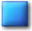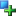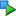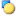##Image_Graph_Axis_Y

Description

Class for storing x-axis settings

Based upon the the common attributes/methods provided by the Image_Graph_Axis-class this one addresses all features which are needed for the y-axis.

Located in /Graph/Elements.php (line 696)

```Image_Graph_Base
|
--Image_Graph_Axis
|
--Image_Graph_Axis_Y```
Method SummaryImage_Graph_Axis_Y &Image_Graph_Axis_Y ()void setAxistype (mixed \$type)void valueToPixelAbsolute (int/float \$value)void valueToPixelRelative (float \$value)
Variables

#### Inherited Variables

Inherited from Image_Graph_AxisMethodsConstructor Image_Graph_Axis_Y (line 726)

Constructor

• access: public
Image_Graph_Axis_Y &Image_Graph_Axis_Y ()setAxistype (line 744)

Set type of axis

This function will allow you to change the type of axis/scale used on this axis. Note that currently only a linear y-axis is supported, so this function is mainly provided for API-completeness and will become useful as soon as other types are implemented.

• see: \$_axistype
• access: public
void setAxistype (mixed \$type)
• mixed \$type: use constants IMAGE_GRAPH_AXISTYPE_*; currently only IMAGE_GRAPH_AXISTYPE_LINEAR supportedvalueToPixelAbsolute (line 788)

Calculate absolute position for a value on the axis

The value returned by this function is in pixel-coordinates and absolute to the upper-left corner of the image canvas.

• access: public
void valueToPixelAbsolute (int/float \$value)
• int/float \$value: data valuevalueToPixelRelative (line 761)

Calculate relative position for a value on the axis

The value returned by this function is in pixel-coordinates and relative to the upper-left corner of the drawing-area of the graph.

• access: public
void valueToPixelRelative (float \$value)
• float \$value: data value

#### Inherited Methods

Inherited From Image_Graph_AxisImage_Graph_Axis::Image_Graph_Axis()Image_Graph_Axis::setBounds()Image_Graph_Axis::setNumbercolor()Image_Graph_Axis::setNumberformat()Image_Graph_Axis::setTicksAutoSteps()Image_Graph_Axis::setTickSize()Image_Graph_Axis::setTicksMajor()Image_Graph_Axis::setTicksMinor()Image_Graph_Axis::setTickStyle()

Inherited From Image_Graph_BaseImage_Graph_Base::setColor()Image_Graph_Base::setFontOptions()Image_Graph_Base::setSpacer()Image_Graph_Base::setText()

Documentation generated on Sat, 21 Feb 2004 23:28:03 +0100 by phpDocumentor 1.3.0RC2